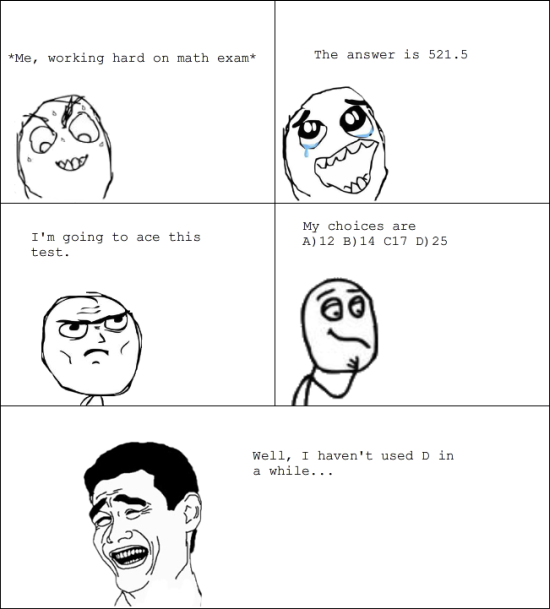An algebra problem by Aneesh Kundu

Algebra Level 5Solve the following system $xy^2=15x^2+17xy+15y^2$

$x^2y=20x^2+3y^2$

$x\not =0, y\not =0$

Enter your answer as the product of all possible distinct complex values of $x$ and $y$.

×